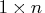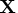Previous Page | Next Page

 Language Reference

## LOC Function

finds nonzero elements of a matrix

LOC( matrix)

where matrix is a numeric matrix or literal.

The LOC function creates arow vector, whereis the number of nonzero elements in the argument. Missing values are treated as zeros. The values in the resulting row vector are the locations of the nonzero elements in the argument (in row-major order, like subscripting). For example, consider the following statements:

```
a={1 0 2 3 0};
b=loc(a);
```
Because the first, third, and fourth elements ofare nonzero, these statements result in the following row vector:
```
B             1 row       3 cols    (numeric)

1         3         4
```

If every element of the argument vector is 0, the result is empty; that is,has zero rows and zero columns.

The LOC function is useful for subscripting parts of a matrix that satisfy some condition.

For example, suppose you want to create a matrixcontaining the rows ofthat have a positive element in the diagonal of. Specify the following statements:

```
x={1  1  0,
0 -2  2,
0  0  3};
y=x[loc(vecdiag(x)>0),];
```
Because the first and third rows ofhave positive elements on the diagonal of, the matrixis as follows:
```
Y             2 rows      3 cols    (numeric)

1         1         0
0         0         3
```

The following example selects all positive elements of a column vector:

```
a={0,
-1,
2,
0};
y=a[loc(a>0),];
```
The resulting output is as follows:
```
Y             1 row       1 col     (numeric)

2
```Previous Page | Next Page | Top of Page